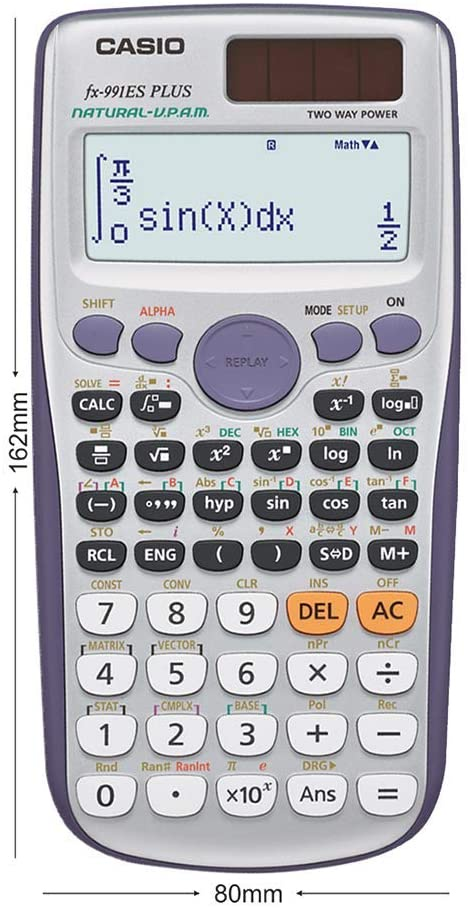Sign in# Tips and tricks about the calculator you may not know

The calculator (CASIO FX-991ES PLUS ) first all let us look at how to clear the calculator memory

1 press shift and 9 the result will be clear? 1 setup 2 memory 3 all

If you are resetting all, press 3 (all) Reset all?

Press (=): yes and (AC): cancel

You can also make the calculator normal,

press shift, press mode, press 8, press 1or2 but 2 is preferable

You can also recall your last answer if you have off the calculator for sometimes,just press answer key and press equal (=)

How to solve linear equation e.g 2x-3=9

STEPS

press 2, press alpha and bracket close, press minus (-) press 3, press shift, press CALC, press 9, press shift press CALC Press equal (=) for the answer

How to convert degree to radian, e.g 90 degree

Press shift, press mode, press 4(rad),enter the angle (90), Press[=], press shift, press answer key, press 1, press (=) for the answer

How to store numbers

Steps involved are

Calculate the equation in the calculator, press shift (a small s will appear on the screen), press RCL button,( STO will be written above it)

Press any of the following A(-), B(•` ``), C(hyp), D(sin), E(cos),F(tan).

The screen will show the with an arrow pointing at the at the answer (eg. Ans=A)

Content created and supplied by: Gafazz (via Opera News )

AC